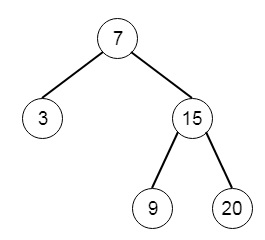# Binary Search Tree Iterator in C++

C++Server Side ProgrammingProgramming

#### C in Depth: The Complete C Programming Guide for Beginners

45 Lectures 4.5 hours

#### Practical C++: Learn C++ Basics Step by Step

Most Popular

50 Lectures 4.5 hours

#### Master C and Embedded C Programming- Learn as you go

66 Lectures 5.5 hours

Suppose we want to make one iterator for binary tree. There will be two methods. The next() method to return the next element, and hasNext() method to return Boolean value, that will indicate that the next element is present or not. So if the tree is like −And the sequence of function calls are [next(), next(), hasNext(), next(), hasNext(),next(), hasNext(),next(), hasNext()]. The output will be [3,7,true,9,true,15,true,20,false]

To solve this, we will follow these steps −

• There are two methods next and hasNext,
• The next() method will be like −
• curr := stack top element, and pop top element
• if right of curr is not null, then push inorder successor from the right of node
• return value of current
• The hasNext() method will be like −
• return true, when stack is not empty, otherwise false.

Let us see the following implementation to get better understanding −

## Example

Live Demo

#include <bits/stdc++.h>
using namespace std;
class TreeNode{
public:
int val;
TreeNode *left, *right;
TreeNode(int data){
val = data;
left = right = NULL;
}
};
void insert(TreeNode **root, int val){
queue<TreeNode*> q;
q.push(*root);
while(q.size()){
TreeNode *temp = q.front();
q.pop();
if(!temp->left){
if(val != NULL)
temp->left = new TreeNode(val);
else
temp->left = new TreeNode(0);
return;
} else {
q.push(temp->left);
}
if(!temp->right){
if(val != NULL)
temp->right = new TreeNode(val);
else
temp->right = new TreeNode(0);
return;
}else{
q.push(temp->right);
}
}
}
TreeNode *make_tree(vector<int> v){
TreeNode *root = new TreeNode(v);
for(int i = 1; i<v.size(); i++){
insert(&root, v[i]);
}
return root;
}
class BSTIterator {
public:
stack <TreeNode*> st;
void fillStack(TreeNode* node){
while(node && node->val != 0){
st.push(node);
node=node->left;
}
}
BSTIterator(TreeNode* root) {
fillStack(root);
}
/** @return the next smallest number */
int next() {
TreeNode* curr = st.top();
st.pop();
if(curr->right && curr->right->val != 0){
fillStack(curr->right);
}
return curr->val;
}
/** @return whether we have a next smallest number */
bool hasNext() {
return !st.empty();
}
};
main(){
vector<int> v = {7,3,15,NULL,NULL,9,20};
TreeNode *root = make_tree(v);
BSTIterator ob(root);
cout << "Next: " << ob.next() << endl;
cout << "Next: " << ob.next() << endl;
cout << ob.hasNext() << endl;
cout << "Next: " << ob.next() << endl;
cout << ob.hasNext() << endl;
cout << "Next: " << ob.next() << endl;
cout << ob.hasNext() << endl;
cout << "Next: " << ob.next() << endl;
cout << ob.hasNext() << endl;
}

## Input

BSTIterator ob(root);
ob.next()
ob.next()
ob.hasNext()
ob.next()
ob.hasNext()
ob.next()
ob.hasNext()
ob.next()
ob.hasNext()

## Output

Next: 3
Next: 7
1
Next: 9
1
Next: 15
1
Next: 20
0